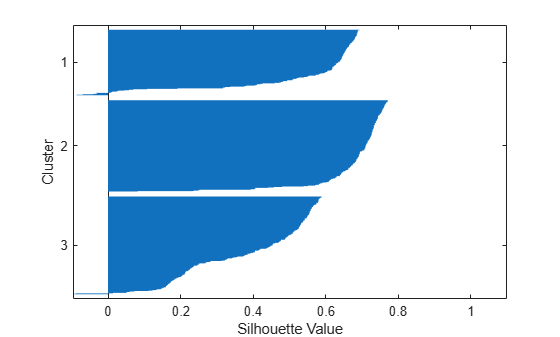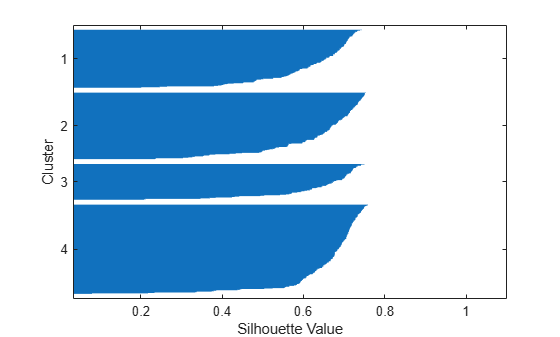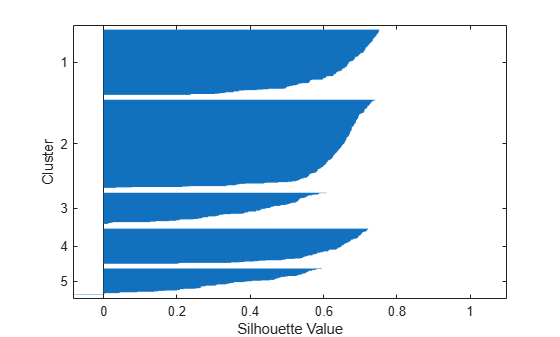Documentation

### This is machine translation

Mouseover text to see original. Click the button below to return to the English version of the page.

## k-Means Clustering

### Introduction to k-Means Clustering

k-means clustering is a partitioning method. The function `kmeans` partitions data into k mutually exclusive clusters, and returns the index of the cluster to which it has assigned each observation. Unlike hierarchical clustering, k-means clustering operates on actual observations (rather than the larger set of dissimilarity measures), and creates a single level of clusters. The distinctions mean that k-means clustering is often more suitable than hierarchical clustering for large amounts of data.

`kmeans` treats each observation in your data as an object having a location in space. It finds a partition in which objects within each cluster are as close to each other as possible, and as far from objects in other clusters as possible. You can choose from five different distance metrics, depending on the kind of data you are clustering.

Each cluster in the partition is defined by its member objects and by its centroid, or center. The centroid for each cluster is the point to which the sum of distances from all objects in that cluster is minimized. `kmeans` computes cluster centroids differently for each distance metric, to minimize the sum with respect to the measure that you specify.

You can control the details of the minimization using several optional input parameters to `kmeans`, including ones for the initial values of the cluster centroids, and for the maximum number of iterations. By default, `kmeans` uses the k-means++ algorithm for cluster center initialization and the squared Euclidean metric to determine distances.

### Create Clusters and Determine Separation

The following example explores possible clustering in four-dimensional data by analyzing the results of partitioning the points into three, four, and five clusters.

### Note

Because each part of this example generates random numbers sequentially, i.e., without setting a new state, you must perform all steps in sequence to duplicate the results shown. If you perform the steps out of sequence, the answers will be essentially the same, but the intermediate results, number of iterations, or ordering of the silhouette plots may differ.

```rng default % For reproducibility load kmeansdata size(X)```
```ans = 1×2 560 4 ```

Even though these data are four-dimensional, and cannot be easily visualized, `kmeans` enables you to investigate whether a group structure exists in them. Call `kmeans` with `k`, the desired number of clusters, equal to `3`. For this example, specify the city block distance metric, and use the default k-means++ algorithm for cluster center initialization.

`idx3 = kmeans(X,3,'Distance','cityblock');`

To get an idea of how well-separated the resulting clusters are, you can make a silhouette plot using the cluster indices output from `kmeans`. The silhouette plot displays a measure of how close each point in one cluster is to points in the neighboring clusters. This measure ranges from +1, indicating points that are very distant from neighboring clusters, through 0, indicating points that are not distinctly in one cluster or another, to -1, indicating points that are probably assigned to the wrong cluster. `silhouette` returns these values in its first output.

```figure [silh3,h] = silhouette(X,idx3,'cityblock'); h = gca; h.Children.EdgeColor = [.8 .8 1]; xlabel 'Silhouette Value' ylabel 'Cluster'```From the silhouette plot, you can see that most points in the second cluster have a large silhouette value, greater than 0.6, indicating that the cluster is somewhat separated from neighboring clusters. However, the third cluster contains many points with low silhouette values, and the first and third contain a few points with negative values, indicating that those two clusters are not well separated.

### Determine the Correct Number of Clusters

Increase the number of clusters to see if `kmeans` can find a better grouping of the data. This time, use the `'Display'` name-value pair argument to print information about each iteration.

`idx4 = kmeans(X,4, 'Distance','cityblock','Display','iter');`
``` iter phase num sum 1 1 560 1792.72 2 1 6 1771.1 Best total sum of distances = 1771.1 ```

A silhouette plot for this solution indicates that these four clusters are better separated than the three in the previous solution.

```figure [silh4,h] = silhouette(X,idx4,'cityblock'); h = gca; h.Children.EdgeColor = [.8 .8 1]; xlabel 'Silhouette Value' ylabel 'Cluster'```A more quantitative way to compare the two solutions is to look at the average silhouette values for the two cases.

`cluster3 = mean(silh3)`
```cluster3 = 0.5352 ```
`cluster4 = mean(silh4)`
```cluster4 = 0.6400 ```

Finally, try clustering the data using five clusters.

```idx5 = kmeans(X,5,'Distance','cityblock','Replicates',5); figure [silh5,h] = silhouette(X,idx5,'city'); h = gca; h.Children.EdgeColor = [.8 .8 1]; xlabel 'Silhouette Value' ylabel 'Cluster'````mean(silh5)`
```ans = 0.5266 ```

This silhouette plot indicates that this is probably not the right number of clusters, since two of the clusters contain points with mostly low silhouette values. Without some knowledge of how many clusters are really in the data, it is a good idea to experiment with a range of values for `k`.

### Avoid Local Minima

Like many other types of numerical minimizations, the solution that `kmeans` reaches often depends on the starting points. It is possible for `kmeans` to reach a local minimum, where reassigning any one point to a new cluster would increase the total sum of point-to-centroid distances, but where a better solution does exist. However, you can use the `'Replicates'` name-value pair argument to overcome that problem.

For four clusters, specify five replicates, and use the `'Display'` name-value pair argument to print out the final sum of distances for each of the solutions.

```[idx4,cent4,sumdist] = kmeans(X,4,'Distance','cityblock',... 'Display','final','Replicates',5);```
```Replicate 1, 4 iterations, total sum of distances = 1771.1. Replicate 2, 2 iterations, total sum of distances = 1771.1. Replicate 3, 5 iterations, total sum of distances = 2303.45. Replicate 4, 4 iterations, total sum of distances = 2303.45. Replicate 5, 6 iterations, total sum of distances = 1771.1. Best total sum of distances = 1771.1 ```

In two of the five replications, `kmeans` found a local (nonglobal) minimum. Since each of these five replicates begin from a different randomly selected set of initial centroids, sometimes `kmeans` finds more than one local minimum. However, the final solution that `kmeans` returns is the one with the lowest total sum of distances, over all replicates.

`sum(sumdist)`
```ans = 1.7711e+03 ```